For a full list of BASHing data blog posts see the index page.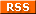# An AWK histogram with scaling

It's not hard to build a frequency-of-occurrence histogram with AWK, but scaling the histogram bars is a little bit trickier. By "scaling" I mean setting the longest bar to a defined character length in the terminal, and adjusting the lengths of all the shorter bars proportionally.

I wanted a scaled histogram so I could visually compare the lengths of data items (number of characters) in a tab-separated field. It's one of the ways I look for truncation in the field, because there are sometimes suspicious bulges or spikes in frequency at 50, 80, 100, 200 or 255 characters. This suggests that some of the data items have come from a database whose fields had character limits, and data items at these bulges need checking.

To demonstrate I'll use field 22 in the TSV "oc". There are no truncations in the field, but it's a handy example. Here are the frequencies for the number of characters in field 22:

awk -F"\t" '{a[length(\$22)]++} END {for (i in a) print i FS a[i]}' oc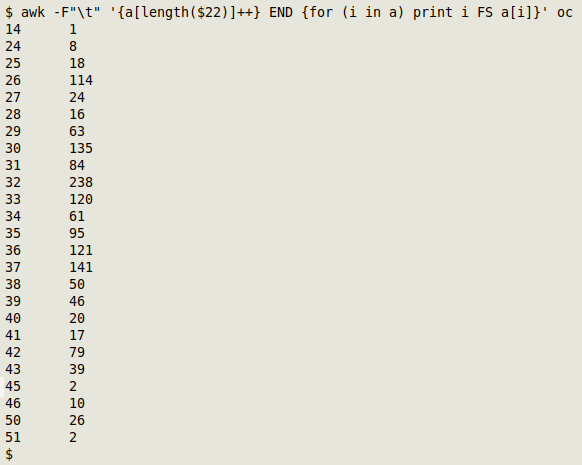So the length of field 22 varies from 14 to 51 characters, and there's a distinct bulge at 32 characters, with 238 occurrences.

And here's a histogram of the length frequencies, scaled so that the longest bar is 50 "*" characters long:

awk -F"\t" '{l=length(\$22); a[l]++; if (a[l]>max) max=a[l]} END {printf("Length\tFrequency"); for (i in a) {printf("\n%s\t%s\t",i,a[i]); for (j=0;j<(int(a[i]*(50/max)));j++) printf("*")} print ""}' oc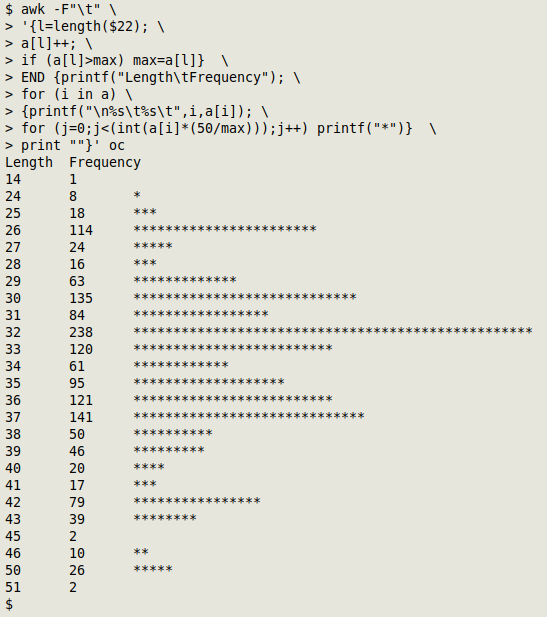awk -F"\t"
The file is a TSV, so the field separator is set to the tab character.

l=length(\$22)
For clarity, I put the length of the desired field into an AWK variable, "l".

a[l]++
Field 22 lengths are put into an array "a". The array is indexed by those lengths, and the array value is the number of times each length occurs.

if (a[l]>max) max=a[l]
This finds the maximum field 22 length as AWK processes the file line by line.

END
Line-by-line processing done, AWK now does something with the information it's gathered, namely an array with lengths and their frequencies, and a maximum length.

printf("Length\tFrequency")
The first task is to printf headers for field length and length frequency. Note that there's no newline specified; newlines are added in the next printf command, at the beginning of each line.

for (i in a)
AWK will loop through the array "a" item by item and do two jobs. The two jobs are enclosed within curly brackets after the for loop is defined.

printf("\n%s\t%s\t",i,a[i])
The first job is to printf a newline, the field length (i), a tab, the number of times that field length occurred (a[i]) and a final tab.

for (j=0;j<(int(a[i]*(50/max)));j++) printf("*")
The second job is to printf "*" a certain number of times. The number runs in a for loop up to the integer value of the length frequency reduced by (in this case) a factor of 50/238. That's the scaling trick. If the integer value is less than 1, nothing gets printed.

print ""
With this final instruction, AWK prints a new line and exits, returning us to the prompt on a new line.

I've modified the command and saved it in the shell function "histlen", which takes three arguments: filename, number of field to be checked, and "threshold". That last parameter allows me to exclude blank data items (l=0) or data items with short, high-frequency lengths, which might otherwise hijack the "max" variable and make bulges at higher lengths less obvious.

In the modified command, AWK will only process lines where the selected field has a length greater than the threshold (condition l>threshold). The AWK output is piped to less -X, because the fields I check often have more than one terminal-page-ful of data item lengths.

histlen() { awk -F"\t" -v fld="\$2" -v threshold="\$3" '{l=length(\$fld)} l>threshold {a[l]++; if (a[l]>max) max=a[l]} END {printf("Length\tFrequency"); for (i in a) {printf("\n%s\t%s\t",i,a[i]); for (j=0;j<(int(a[i]*(50/max)));j++) printf("*")} print ""}' "\$1" | less -X; }

In the real-world file "example" there's truncation in field 21 at 50 characters, and many blanks. Compare the histograms, below, with and without a threshold set at zero: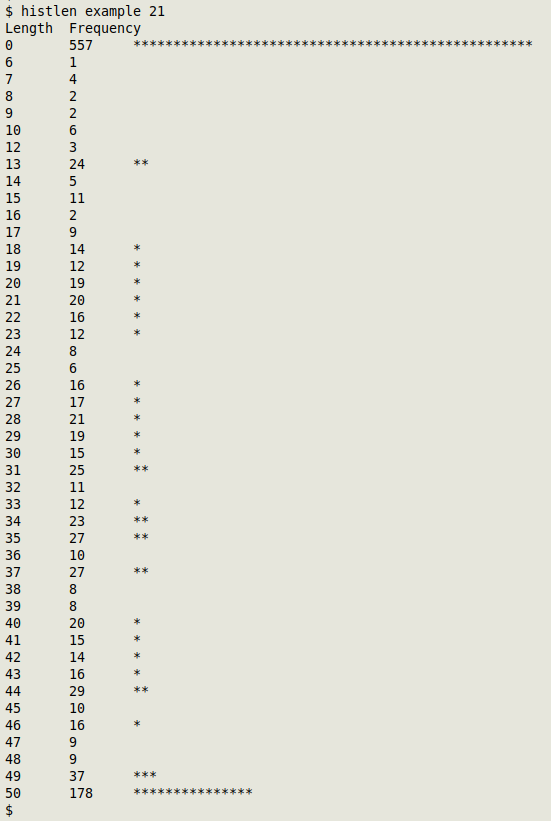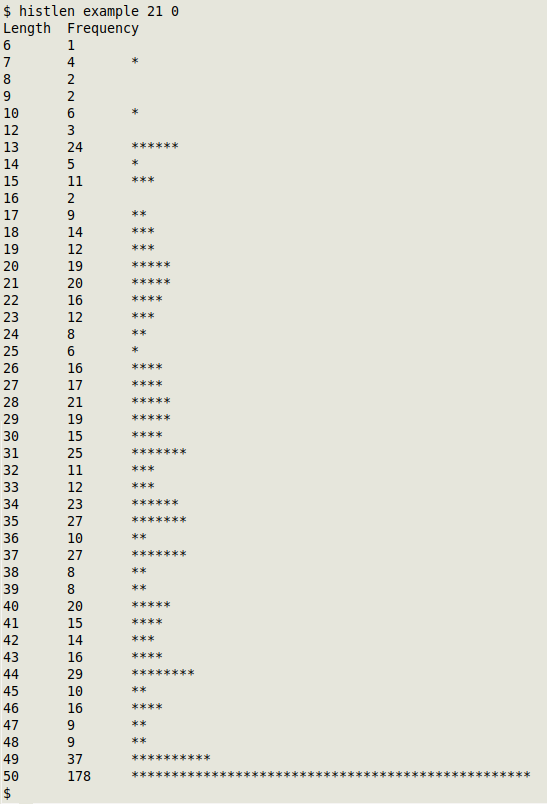Update. Reader Håkan Winbom recommends adding PROCINFO["sorted_in"] = "@ind_num_asc" to the command, customarily in a BEGIN statement, to ensure that GNU AWK traverses the array "a" in numerical order of the index, which is field length. This is a good idea, although with the GNU AWK version I'm using (4.2.1) this sorting is the default: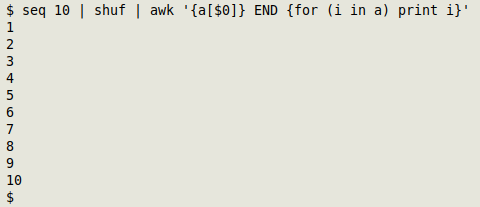Last update: 2021-09-23
The blog posts on this website are licensed under a